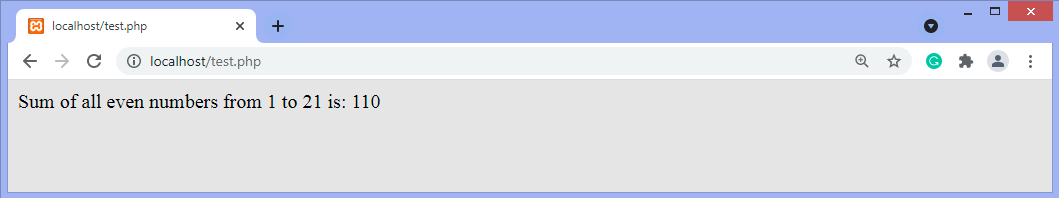Q:

# Write a program to find the sum of even numbers

In this article, we will discuss the program to find the sum of even numbers in different programming languages.

But before writing the programs, let's first see a brief description of even numbers and the formula to find the sum of even numbers.

### Even numbers

Any integer number that is completely divided by 2 is known as the even number. All the even numbers must be divided by 2. To check whether the given integer number is an odd number or an even number; we have to check the number's last digit. The even numbers always end with the last digit of 0, 2, 4, 6, and 8.

## Sum of even numbers

It is easy to find the sum of even numbers from 2 to infinity. It can be obtained by using the formula for finding the sum of natural numbers or by using the arithmetic progression.

We can use the formula n(n+1) to find the sum of even numbers, where n is any natural number. For instance, if we have to find the sum of the first four even numbers 2, 4, 6, and 8, the value of n will be 4.

i.e. sum of first four even numbers = 2 + 4 + 6 + 8 = 20

or, = 4(4+1) = 4 * 5 = 20 (n = 4)

If we have to find the sum of first three even numbers that are 2, 4, and 6, then the value of n will be 3. So,

3(3+1) = 12

Or, 2 + 4 + 6 = 12

### Programs to find the sum of even numbers

Program: Write a program to find the sum of even numbers in C language.

``````#include <stdio.h>
int main()
{
int i, n, sum=0;
printf("Enter any number: ");
scanf("%d", &n);
for(i=2; i<=n; i+=2)
{
sum += i;
}
printf("Sum of all even numbers from 1 to %d: %d", n, sum);
return 0;
}  ``````

Output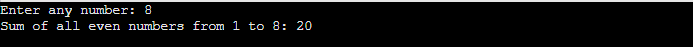Program: Write a program to find the sum of even numbers in the C# language.

``````using System;
public class Jtp
{
static int fun (int n)
{
int i, sum = 0;
for (i = 2; i <= n; i += 2)
{
sum += i;
}
return sum;
}

public static void Main ()
{
int n;
Console.Write ("Enter any number: ");
Console.WriteLine ("Sum of all even numbers from 1 to " + n + " is: " + fun (n));
}
}  ``````

Output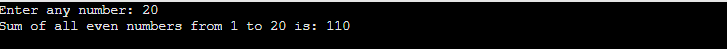Program: Write a program to find the sum of even numbers in JavaScript.

``````<!DOCTYPE html>
<html>
<body>
<script>
function fun(n)
{
let i, sum = 0;
for (i = 2; i <= n; i+=2) {
sum += i;
}
return sum;
}
let n = prompt("Enter any number: ");
document.write("Sum of all even numbers from 1 to " + n + " is: " + fun(n));
</script>
</body>
</html>  ``````

Output

After the execution of the above code, the output will be -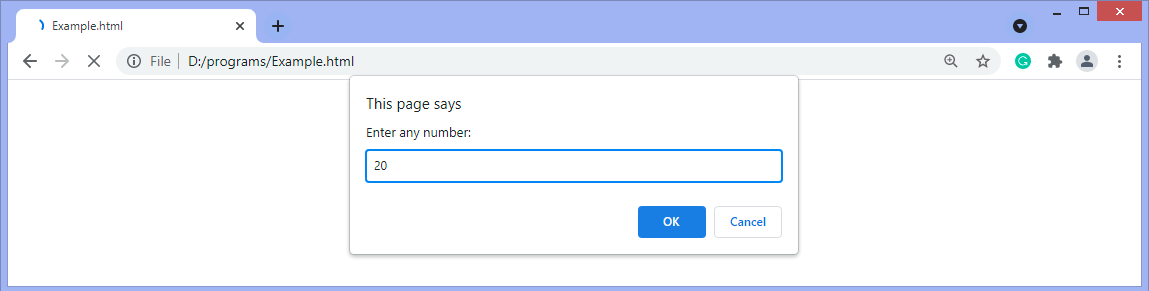After entering the value and clicking on OK, the output will be -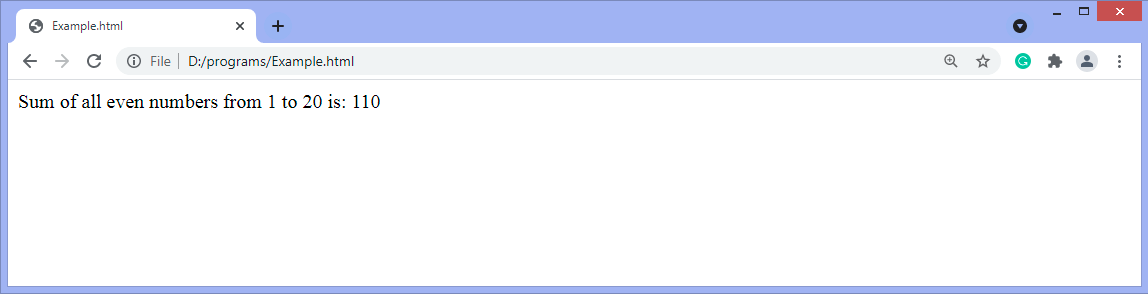Program: Write a program to find the sum of even numbers in Java.

``````import java.util.*;
import java.lang.*;

public class Jtp{
static int fun(int n)
{
int i, sum = 0;
for (i = 2; i <= n; i+=2) {
sum += i;
}
return sum;
}
public static void main(String argc[])
{
System.out.println("Enter any number: ");
Scanner sc = new Scanner(System.in);
int n = sc.nextInt();
System.out.println("Sum of all even numbers from 1 to " + n + " is: " + fun(n));
}

}  ``````

OutputProgram: Write a program to find the sum of even numbers in python.

``````def fun(n):
sum = 0
i = 2
while i <= n:
sum += i
i = i + 2
return sum

n = int(input('Enter any number: '))
print("Sum of all even numbers from 1 to", n , "is: " , fun(n))  ``````

Output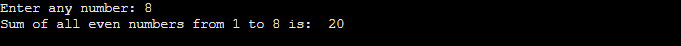Program: Write a program to find the sum of even numbers in PHP.

``````<?php
function fun(\$n)
{
\$sum = 0;
for (\$i = 2; \$i <= \$n; \$i+=2) {
\$sum += \$i;
}
return \$sum;
}
\$n = 21;
echo "Sum of all even numbers from 1 to ". \$n . " is: " . fun(\$n);
?>  ``````

Output